Courses

# Test: Analog Communication - 2

## 20 Questions MCQ Test GATE ECE (Electronics) 2022 Mock Test Series | Test: Analog Communication - 2

Description
This mock test of Test: Analog Communication - 2 for Electronics and Communication Engineering (ECE) helps you for every Electronics and Communication Engineering (ECE) entrance exam. This contains 20 Multiple Choice Questions for Electronics and Communication Engineering (ECE) Test: Analog Communication - 2 (mcq) to study with solutions a complete question bank. The solved questions answers in this Test: Analog Communication - 2 quiz give you a good mix of easy questions and tough questions. Electronics and Communication Engineering (ECE) students definitely take this Test: Analog Communication - 2 exercise for a better result in the exam. You can find other Test: Analog Communication - 2 extra questions, long questions & short questions for Electronics and Communication Engineering (ECE) on EduRev as well by searching above.
*Answer can only contain numeric values
QUESTION: 1

Solution:
QUESTION: 2

### A 100MHz carrier is frequency modulated by 10 KHz wave. For a frequency deviation of 50 KHz, calculate the modulation index of the FM signal.

Solution:

Carrier frequency fc = 100MHz
Modulating frequency fm = 10 KHz
Frequency deviation Δf = 500 KHz
Modulation index of FM signal is given by
mf = Δf/fm
= 500 * 103/ 10 * 103
= 50

QUESTION: 3

### Consider a system shown in the figure-1. Let X(f) and Y(f) represent the Fourier transforms of x(t) and y(t) respectively. The ideal HPF has cutoff frequency of 10 KHZ. X(f) is shown in the figure-2. The positive frequency where Y(f) has spectral peaks are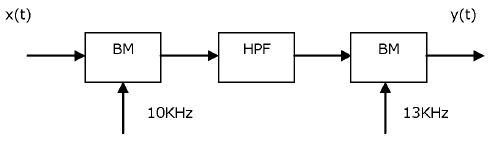​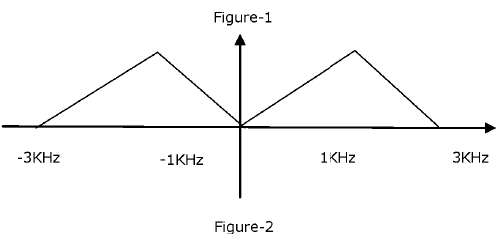Solution:

2 KHz S. 24 KHz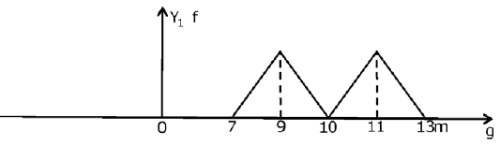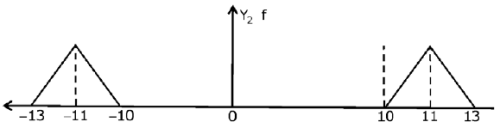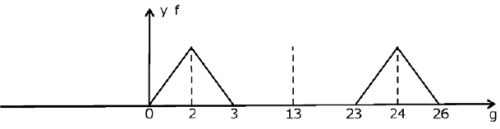QUESTION: 4

Three message signals each band limited to 10 kHz, 10 kHz, and 5 KHz are transmitted through a channel using frequency division multiplexing. The modulation techniques used are any, D513-SC, SSB respectively. The guard band is 2 KHz. Band width of the channel is

Solution:

BW after AM modulation = 2 x 10 = 20 KHz

BW after DSS-SC modulation = 2 x10 = 20 KHz BW after SSB modulation = 5 KHz

Guard band width = 2 x 2k=4 k

∴ Total BW after frequency multiplexing = 49 KHz

QUESTION: 5

A signal '4 t is band limited to the frequency range 0 to fn. The signal frequency is translated by multiplying it by the signal V, t —cos2πfct . The value of fc such that the bandwidth of the translated signal is 1% of the carrier frequency fc will be

Solution:

BW after multiplexing =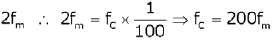*Answer can only contain numeric values
QUESTION: 6

The peak amplitude of an AM signal for tone modulation with carrier having maximum amplitude as 5 V, varies from 2 to 8 V then the sideband power is  _________________________ (W)

Solution: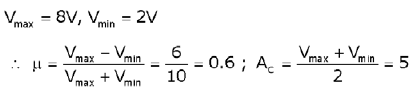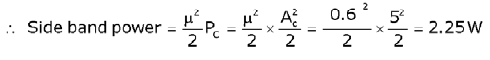QUESTION: 7

Consider a frequency multiplier shown in the figure below.ss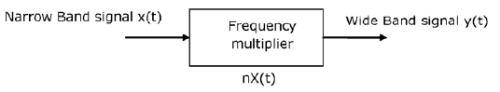A NBFM signalX Mal t with f, = 200KHz and f, ranges 50 Hz to 150 KHz is given as an input to frequency multiplier. The Maximum Frequency deviation of the NBFM is 25 Hz. The maximum frequency deviation at the output is 75 KHz.

Q. The required frequency multiplication n is

Solution: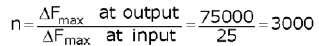QUESTION: 8

Consider a frequency multiplier shown in the figure below.ssA NBFM signalX Mal t with f, = 200KHz and f, ranges 50 Hz to 150 KHz is given as an input to frequency multiplier. The Maximum Frequency deviation of the NBFM is 25 Hz. The maximum frequency deviation at the output is 75 KHz.

Q. The carrier frequency at the output is

Solution:
QUESTION: 9

SSB modulation may be viewed as a hybrid form of amplitude modulation and frequency modulation. Assuming a message signal m(t) = sin t and a carrier frequency 'fc', the instantaneous frequency for the lower side band is

Solution:

For SSB modulation, the modulated wave is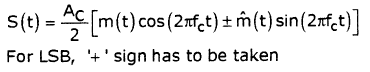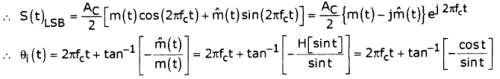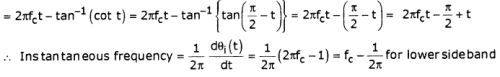QUESTION: 10

Given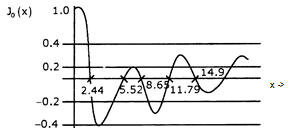In a certain experimental conducted with fm = 1 kHz and increasing Am(starting from 0 volts), it is found that the carrier component of the FM signal is reduced to zero for the first time when Am = 2 volts.

The frequency sensitivity of the modulator is

Solution:

When Am = 2V, carrier component = 0

ie. J0 (03) = 0 for the 1st time

From the curve, corresponding (3=2.44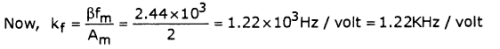*Answer can only contain numeric values
QUESTION: 11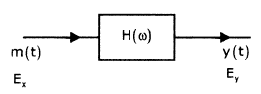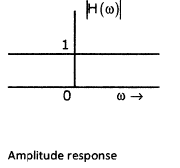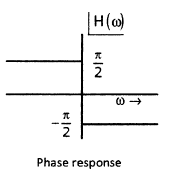If Ex and Ey represent the energies of m(t) and y(t) respectively, then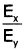_______________

Solution:

Mh (ω) = -jM (6)) sgn(ω), (The given system is a Hilbert transformer) The π of a Hilbert transformer is

H (ω) = -jsgn (ω)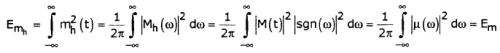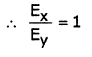QUESTION: 12

Determine the spectrum of y(t) in the system shown below.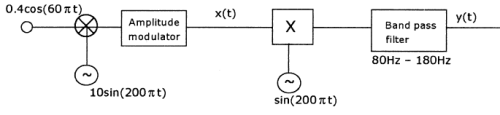Solution:

Output of AM Modulator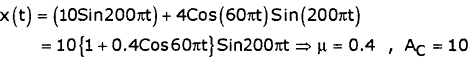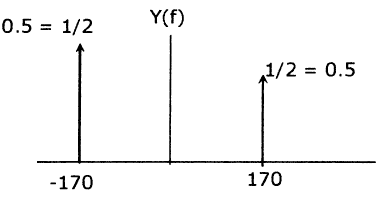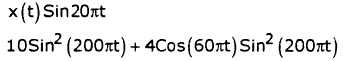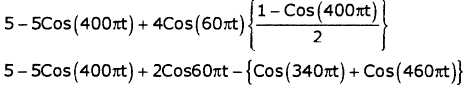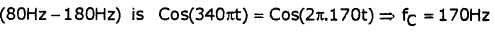QUESTION: 13

Consider the scheme shown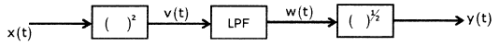If x (t) = m (t) cos (coct) then y (t) is

Solution: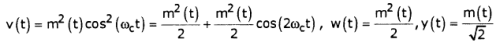QUESTION: 14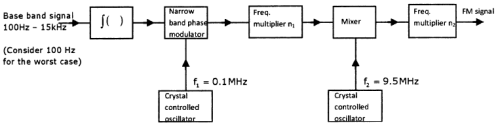The above schematic generates FM signal as a wideband frequency modulator

using the indirect method. The output FM signal is characterized by fc = 100 MHz, Δf = 75kHz (min). If the phase modulator has a modulation index of β= 0.3 radians, then n1, n2 are respectively

Solution:

The lowest modulation frequency i.e.,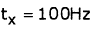Produces a frequency deviation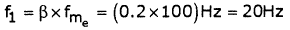The highest modulation frequency ie.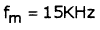Produces a frequency deviation of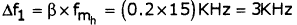Let us take the case of 100Hz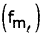as the worst case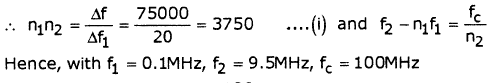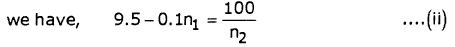Solving (1) & (2) we get,

n1=75 , n2 =50

QUESTION: 15

What is the standard IF frequency for AM receivers?

Solution:

In AM receivers, the standard intermediate frequency is approximately 455 KHz.

QUESTION: 16

Consider a message signal m(t) = 2sin(10007t)

Frequency sensitivity constant Kf=75KHz/V

Q. If amplitude of message signal is doubled, then bandwidth for FM and PM will be

Solution:

Δf = 75x 4 = 300KHz ,

BFM = 2(300 + 0.5) = 601KHz, Δ f= 2 x Kp x 2 x 500 = 10KHz,Bpm = 2(10 + 0.5) = 21KHz

QUESTION: 17

A 20 KW and 1 MHz carrier is frequency modulated by a sinusoidal signal so that the maximum frequency deviation is 50 KHz and the message signal is band limited to 500 KHz. The generated FM signal is amplitude modulated using carrier of 1 GHz.

Q. The bandwidth of the generated signal is

Solution: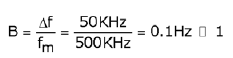It is a narrow band FM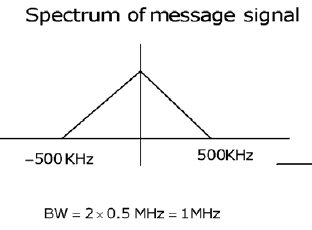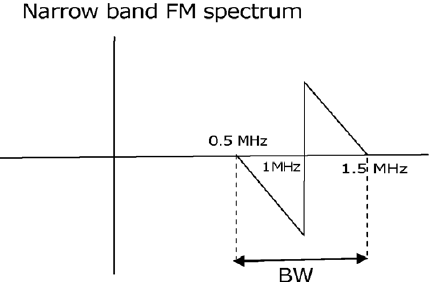QUESTION: 18

A 20 KW and 1 MHz carrier is frequency modulated by a sinusoidal signal so that the maximum frequency deviation is 50 KHz and the message signal is band limited to 500 KHz. The generated FM signal is amplitude modulated using carrier of 1 GHz.

Q. The bandwidth of the generated AM signal is

Solution:

Taking above spectrum as message signal and generate AM signal using carrier 1GHZ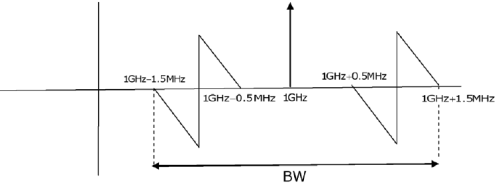BW = 2 1,5 MHz = 3MHz

QUESTION: 19

The input x(t) and the output y(t) of a certain nonlinear channel are related as y(t) = x(t) + 0.001 x2(t)

If frequency spectrum of input signal is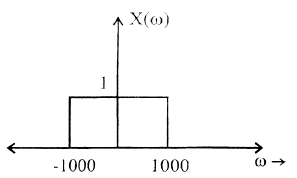Q. The bandwidth of the output signal is

Solution:
QUESTION: 20

The input x(t) and the output y(t) of a certain nonlinear channel are related as y(t) = x(t) + 0.001 x2(t)

If frequency spectrum of input signal isQ. Spectrum of the received signal after appropriate low pass filtering is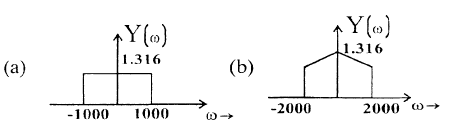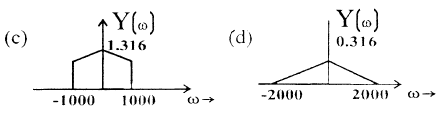Solution: